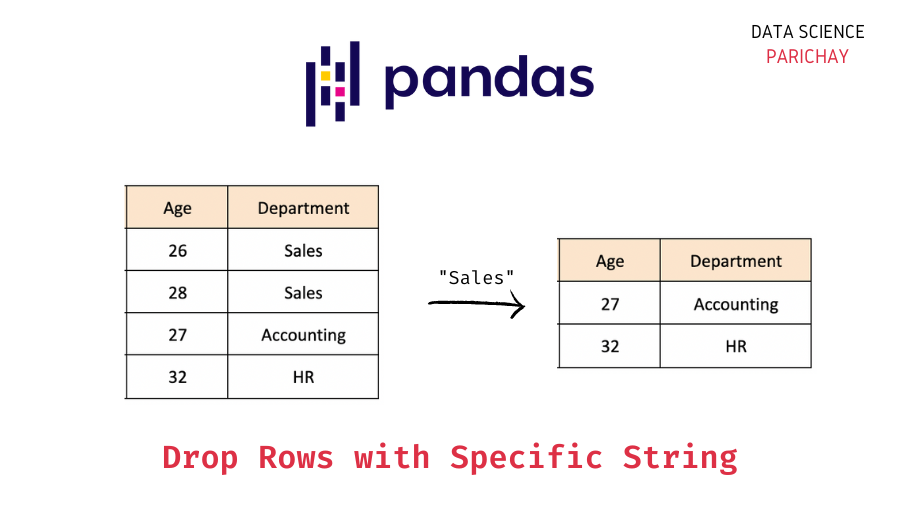# Pandas – Drop Rows that Contain a Specific String

The pandas module in Python comes with a number of built-in functions to help you work with and manipulate tabular data. In this tutorial, we will look at how to drop (or remove) rows that contain a specific string in a given column.

## How to Drop Rows that Contain a Specific String?

You can use the pandas built-in `drop()` function to drop rows from a dataframe. Pass the index of the rows to drop (in our case, the row indices where the given column contains a specific string). It returns the resulting dataframe after dropping the mentioned rows.

The following is the syntax.

```# drop rows that contain a specific string in a given column
df.drop(df[df["col_name"].str.contains("string")].index)```

Here, we use the `.str` accessor on the column “col_name” and check if it contains the string “string”, this results in a boolean mask that we use to filter the dataframe and get the index of the rows to drop which we pass the to `drop()` function.

Note that the `drop()` function does not modify the dataframe in place by default, rather it returns the resulting dataframe.

Alternatively, you can also use boolean filtering to get the same result as above. The idea is to filter the dataframe such it gives us only the rows that do not contain the given string in the mentioned column.

The following is the syntax.

```# drop rows that contain a specific string in a given column
df[df["col_name"].str.contains("string")==False]```

This will give us the rows where the “col_name” column does not contain the string “string”.

📚 Data Science Programs By Skill Level

Introductory

Intermediate ⭐⭐⭐

🔎 Find Data Science Programs 👨‍💻 111,889 already enrolled

Disclaimer: Data Science Parichay is reader supported. When you purchase a course through a link on this site, we may earn a small commission at no additional cost to you. Earned commissions help support this website and its team of writers.

## Examples

Let’s now look at some examples of using the above syntax.

First, we will create a pandas dataframe that we will be using throughout this tutorial.

```import pandas as pd

# cricket team data
data = {
'Team': ['India', 'South Africa', 'Australia', 'Pakistan', 'Sri Lanka', 'West Indies', 'Netherlands', 'Bangladesh','England'],
'Points': [10, 10, 8, 8, 7, 6, 7, 4,8],
'Run Rate': [1.1, 1.3, 0.6, 0.1, 0.9, -0.5, -0.1, -1.0,1.5],
'Group': ['A', 'B', 'A', 'A', 'C', 'B', 'C', 'B','C']
}

# create pandas dataframe
df = pd.DataFrame(data)
# display the dataframe
df```

Output:

Here, we created a dataframe with information about 8 teams played in a cricket tournament. The dataframe has the following columns – “Team”, “Points”, “Run Rate”, and “Group”.

### Example 1: Drop rows that contain a specific string

The following code shows how to drop all rows in the above dataframe that contain “A” in the “Group” column:

```# drop rows that contain a specific string in a given column
df.drop(df[df["Group"].str.contains("A")].index)```

Output:

Here, we get first get the index of rows that contain the string “A” in the “Group” column and then pass these indices to the `drop()` function which drops the rows corresponding to those indices.

### Example 2: Filter out rows that do not contain a specific string

Alternatively, you can just filter out the rows that you don’t want using boolean indexing in pandas dataframes. Here, since we don’t want the rows that contain a specific string in a given column, we will filter out these rows.

Let’s take the same example from above. Remove rows that contain “A” in the “Group” column.

```# drop rows that contain a specific string in a given column
df[df["Group"].str.contains("A")==False]```

Output:

We get the same results as above.

## Summary

In this tutorial, we looked at how to remove rows from a dataframe that contain a specific string in a given column. The following are the methods covered –

• Using the pandas `drop()` function. Pass the indices of the rows to drop.
• By filtering the dataframe using boolean indexing in the dataframe.

You might also be interested in –

••